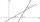# Right

Determine angles of the right triangle with the hypotenuse c and legs a, b, if:Result

α =  64.6 °
β =  25.4 °
γ =  90 °

#### Solution:Leave us a comment of example and its solution (i.e. if it is still somewhat unclear...):Be the first to comment!#### To solve this verbal math problem are needed these knowledge from mathematics:

Looking for help with calculating roots of a quadratic equation? Pythagorean theorem is the base for the right triangle calculator. Most natural application of trigonometry and trigonometric functions is a calculation of the triangles. Common and less common calculations of different types of triangles offers our triangle calculator. Word trigonometry comes from Greek and literally means triangle calculation.

## Next similar examples:

1. ForcesIn point O acts three orthogonal forces: F1 = 20 N, F2 = 7 N and F3 = 19 N. Determine the resultant of F and the angles between F and forces F1, F2 and F3.
2. DiagonalDetermine the dimensions of the cuboid, if diagonal long 53 dm has angle with one edge 42° and with other edge 64°.
3. Trigonometric functionsIn right triangle is: ? Determine the value of s and c: ? ?
4. MastMast has 17 m long shadow on a slope rising from the mast foot in the direction of the shadow angle at angle 9.3°. Determine the height of the mast, if the sun above the horizon is at angle 44°30'.
5. IS triangleCalculate interior angles of the isosceles triangle with base 38 cm and legs 26 cm long.
6. Trapezoid MOThe rectangular trapezoid ABCD with right angle at point B, |AC| = 12, |CD| = 8, diagonals are perpendicular to each other. Calculate the perimeter and area of ​​the trapezoid.
7. ConeCalculate volume and surface area of ​​the cone with diameter of the base d = 15 cm and side of cone with the base has angle 52°.
8. Rotation of the EarthCalculate the circumferential speed of the Earth's surface at a latitude of 61°​​. Consider a globe with a radius of 6378 km.
9. Regular 5-gonCalculate area of the regular pentagon with side 7 cm.
10. Triangle SASCalculate the area and perimeter of the triangle, if the two sides are 51 cm and 110 cm long and angle them clamped is 130 °.
11. RectangleCalculate the length of the side GN and diagonal QN of rectangle QGNH when given: |HN| = 25 cm and angle ∠ QGH = 28 degrees.
12. Rotary coneThe volume of the rotation of the cone is 472 cm3 and angle between the side of the cone and base angle is 70°. Calculate lateral surface area of this cone.
13. PentagonCalculate the area of regular pentagon, which diagonal is u=17.
14. CuboidDetermine the dimensions of cuboid a, b, c; if diagonal d=9 dm has angle with edge a α=55° and has angle with edge b β=58°
15. TriangleTriangle KLM is given by plane coordinates of vertices: K[-12, -11] L[-15, -18] M[-13, -12]. Calculate its area and itsinterior angles.
16. Earth parallelEarth's radius is 6370 km long. Calculate the length parallel of latitude 50°.
17. Angle between linesCalculate the angle between these two lines: ? ?